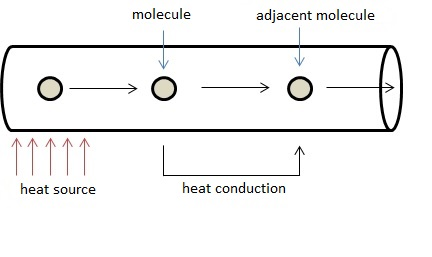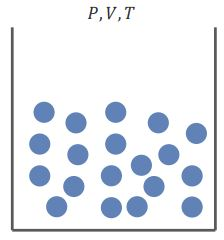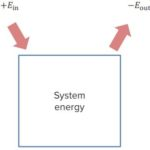Are you more of a visual learner? Check out our online video lectures and start your physics course now for free!Definition of Temperature

T = Temperature [K], [°C]

Temperature is a physical parameter. The following statements are based on the temperature of substances:

• Thermodynamic equilibrium exists if 2 bodies at the same temperature do not exchange heat between them. If they are at different temperatures, heat flows from the warmer to the colder body until an equilibrium is achieved.
• The average kinetic energy of a gas depends directly on its temperature.
• The properties of substances that depend on temperature include thermal expansion, density, and electric resistance.

The basic unit (SI) of temperature is Kelvin [K]. In Europe, it is also common to use °C (1°C = 273.15 K).

The astronomer Anders Celsius defined benchmarks for the Celsius scale. According to Celsius, the boiling point of pure water was 0°C and the melting point was 100°C. The boiling and melting points were only reversed after his death, although he laid the foundation for temperature measurements used currently.

The Zeroth Law: The Concept of TemperatureImage: 0th-Law-Temperature. By Lecturio

Quantities that only depend on the appearance of a system are known as state variables. They only depend on the current state:

• Examples are pressure, temperature, and volume
• Counter-example is ‘the amount of added heat’

Temperature is equivalent for all materials, such that if 2 objects are in thermal equilibrium with a 3rd, they are in equilibrium with each other.

The Absolute Zero

According to its definition, absolute 0 is the lowest temperature that can still be measured. It is at 0 Kelvin (= – 273.15°C). The theory implies that gases get colder with diminishing particle speed. At the absolute 0, the speed of all particles equals 0, suggesting that no negative temperatures can be measured on the absolute temperature scale (Kelvin).

Thermal State Equations for Gases

For ideal gases, the pressure is directly proportional to the temperature. The following laws describe the correlation between 2 parameters while other parameters remain constant:

Law of Boyle-Mariotte

If the pressure of an ideal gas is increased and the temperature and the amount of substance remain constant, the pressure is inversely proportional to the volume:

ρ ∼ 1 / V

Law of Gayle-Lussac

The volume of an ideal gas is proportional to its temperature as long as the amounts of substance and pressure remain constant.

V ∼ T

Amonton’s Law

If an ideal gas is heated, the pressure is increased, and when cooled down, the pressure of the gas declines.

Coefficient of Thermal Expansion

Different substances expand to different degrees under a constant increase in temperature. The phenomenon is known as thermal expansion, which does not occur steadily, and is classified depending on the extent of expansion:

Linear Coefficient of Thermal Expansion

The linear coefficient of thermal expansion is defined as the change in length of a substance in relation to its total length.

 α Coefficient of linear expansion [10-6 / K ] at 20°C L Length [m] ΔL Change of length [m] ΔT Change of temperature [K]

α = 1 / L * ΔL / ΔT

Volume-specific Expansion Coefficient/Cubic Expansion Coefficient

The volume-specific expansion coefficient is defined as the change in volume of a body in relation to its total volume when the temperature is changed by 1 K.

 γ Cubic expansion coefficient [10-3 / K] at 20°C V0 Volume prior to heating [m³] δV Change of volume [m³] δT Change of temperature [K]

γ = 1/ V0 * (δV / δT)

Temperature Measurements

The temperature of a substance can be measured depending on its different characteristics.

Liquid Thermometer

A liquid—usually mercury or dyed alcohol—is placed in a thin tube with an attached scale. The temperature is measured by the changes in volume during heating or cooling of the liquid. The liquid in the thermometer must:

• expand quickly and uniformly across a wide range of temperatures.
• not stick to the sides of the tube.

Water is not appropriate due to the anomalous expansion of water, i.e., if the water is heated from -15°C, it expands but only until 0°C. At this point, it starts to melt and contract up to 4°C. Upon further heating, the water expands uniformly until its boiling point.

Gas Thermometer

A drop of mercury is placed in a thin tube, similar to the liquid thermometer. The tube encloses a small volume of gas, which expands if warmed up or decreases upon cooling down.

Bimetallic Thermometer

The thermal expansion varies depending on the substance. A coiled bimetallic strip consists of 2 different types of metal and is attached to an indicator. When the bimetallic strip is heated, the 2 metals expand at different intensities, which leads to a deflection of the indicator.

Electronic Thermometer/Resistance Thermometer

Electric resistance strongly depends on the temperature, especially in the case of semiconductors. The resistance of a thermistor decreases with increasing temperature. Reduction in resistance leads to an increased current flow, which alters the temperature.

Thermochromism

Thermochromic materials change color or emit light following changes in temperature.

Heat

 Q Heat [J] c specific heat capacity [J/(kg*K)]

An increase in temperature results in an increase in the kinetic energy of the smallest parts. Heating implies energy input. Cooling implies energy extraction.

Heat is a special type of energy. According to the principle of conservation of energy, the inner energy can only be generated via conversion of other types of energy such as mechanic, electric, chemical or nuclear energy.

Remember: Energy cannot be destroyed but only converted from one form of energy into another (see also article Mechanics I).

Heat and inner energy are equal to other forms of energy and at least partially interconvertible.

The absorbed heat of a material is proportional to its mass and to the change in its temperature. The specific heat capacity generally indicates the amount of heat necessary to heat one kilogram of a specific substance by 1 K. A calorimeter such as heat flow calorimeter, heat balance calorimeter or adiabatic calorimeter can be used to measure the specific heat capacity, which is the change of heat within a body and thus the change of inner kinetic energy.

Heat is calculated as:

ΔQ = c * m * ΔT

The heat absorbed or emitted by a body equals the product of specific heat capacity, the mass of the body and the change of temperature.Image: 1st law – energy. By Lecturio

First Law of Energy

The energy in a closed system is conserved. Energy can be added or subtracted to the system but is not lost or gained within a system. The internal energy of a system is expressed as U.

Thermal Output

 P Thermal output [W]

If a heat source provides a specific amount of heat within a specific interval of time, the thermal output is defined by the quotient of heat and time.

P = ΔQ / Δt

Thermal Convection

Heat can be transferred in 3 different ways: conduction, convection, and radiation. In this process, heat is transferred from a hotter body to a colder one.

If a body is heated in one place, the density of the liquid is reduced at that location due to the increase in temperature. Buoyancy raises the warm liquid up and the colder liquid sinks to the bottom, which leads to the transport of water mass carrying heat energy.

Humans continuously exchange heat with their surroundings via four mechanisms.

Conduction (transfer of heat via direct contact)

Heat travels from places at a higher temperature to neighboring (bordering) places at a lower temperature via the transfer of kinetic energy between different molecules. The better the conductor, the more rapid is the heat transfer.

Convection (exchange of heat through a medium such as air or water)

The movement of particles in liquids and gases is also defined as convection, which is an important factor controlling the emission of body heat during thermoregulation. Convection facilitates the transport of blood gases throughout the body with the blood flow.

Heat radiation does not require an intermediate medium to transport thermal energy from a warmer body to a colder one. Heat rays are not only emitted by warm bodies that emit light but also by non-light emitting bodies when their temperature is higher than the temperature of their surroundings. Upon the impact of heat rays, a body at a lower temperature is heated up.

Evaporation (loss of heat through vaporization)

Vaporization occurs via skin in the form of sweat.

The Principle of Thermodynamics

 W work performed [J] H enthalpy [J] U inner energy, has no unit p pressure V volume

The state of a gas is identified depending on the 3 state variables: pressure, volume, and temperature. Change in 2 or all state variables is referred to as a change of state. Each state of a system carries a specific, clearly defined value of inner energy, based on the 1st principle of thermodynamics applies. The input of heat and kinetic energy increases the inner energy of a closed system.

If Q is the amount of heat energy input, W denotes the conducted mechanical work and ΔU represents the change of inner energy, then:

ΔU = Q + W

In the case of ideal gases, mechanical work leads to a change in volume. The input of heat energy results in increased inner energy and volume. The inner energy refers to the total energy within a system. It only depends on the states of pressure, volume, and temperature. The change of inner energy is determined only by initial and final states.

The sum of inner energy and the product of pressure and volume is referred to as enthalpy. The product of pressure and volume equals the amount of displacement.

H = U + (ρ * V)

Thermal Conduction

Since the conduction of heat is a particularly important topic in medicine, this chapter will address the issue in further detail.

The easiest mode of heat transfer is heat conduction. As mentioned earlier, heat transfer occurs from a warmer substance to the colder one.

Heat conduction only occurs inside matter and across a temperature gradient. Different materials display different heat conductivity, which is expressed as the coefficient of thermal conductivity. The coefficient of thermal conductivity is the amount of heat that is transferred per time unit across 2 opposite sides of a cube with an edge length of 1 m and a temperature difference of 1 K, with the other sides of the cube remaining completely impermeable to heat.

It is also possible to express the thermal conductivity of materials with respect to a specific substance, to obtain the relative conductivity.

Metals generally are good heat conductors. Bad heat conductors are gases, wool, paper, and other materials, which are used in thermal insulation.

Different heat therapies are used in medicine, for example, to improve and preserve the function of the locomotor system, to strengthen and relax musculature, improve the muscle tone, or relieve pain.

Learn. Apply. Retain.
Your path to achieve medical excellence.
Study for medical school and boards with Lecturio.